CBSE Class 10 Sample Paper for 2021 Boards - Maths Standard

Class 10
Solutions of Sample Papers for Class 10 Boards

## Parabolas are symmetric about a vertical line known as the Axis of Symmetry . The Axis of Symmetry runs through the maximum or minimum point of the parabola which is called the

Note: Check more Case Based Questions for Polynomials Class 10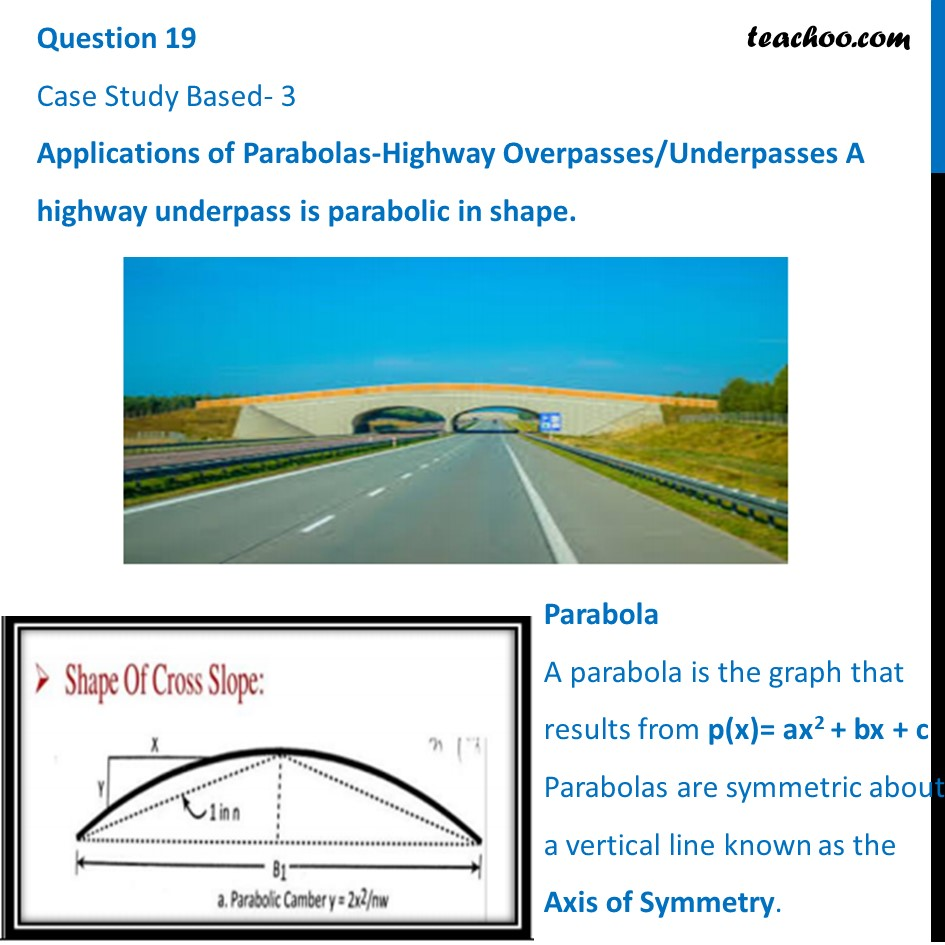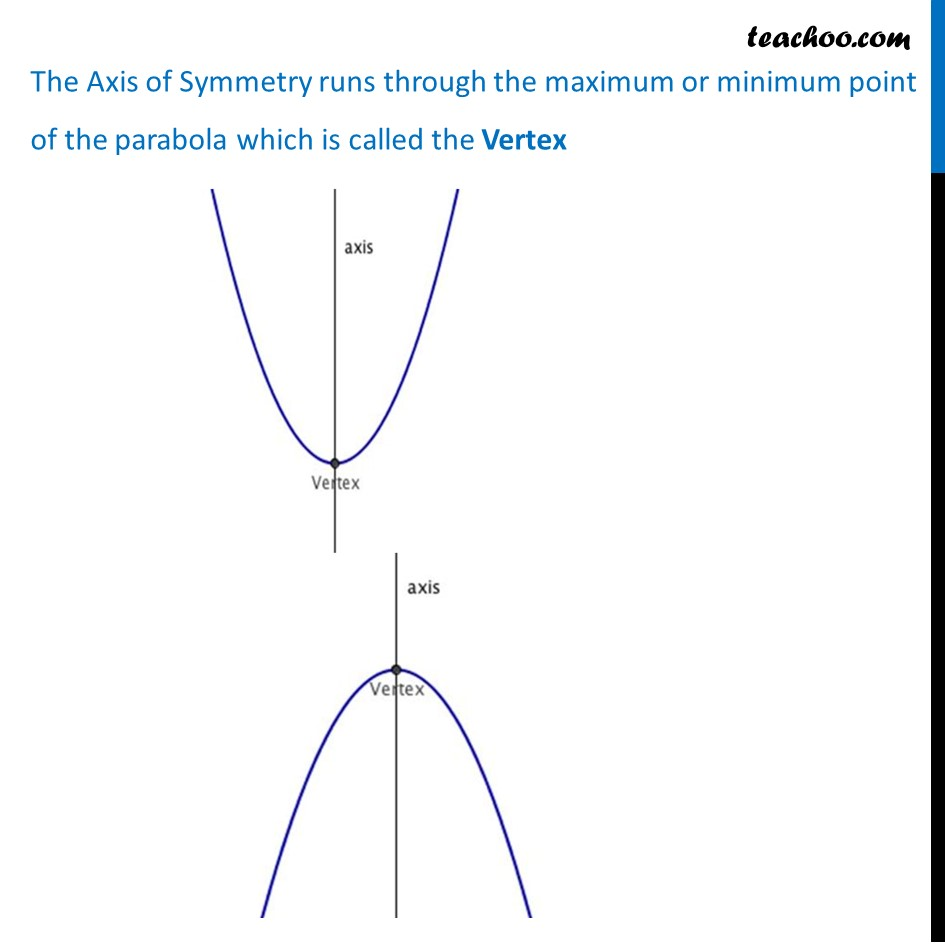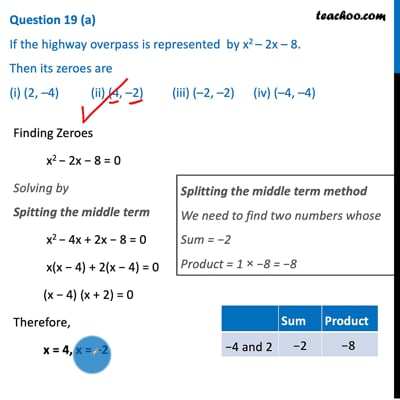This video is only available for Teachoo black users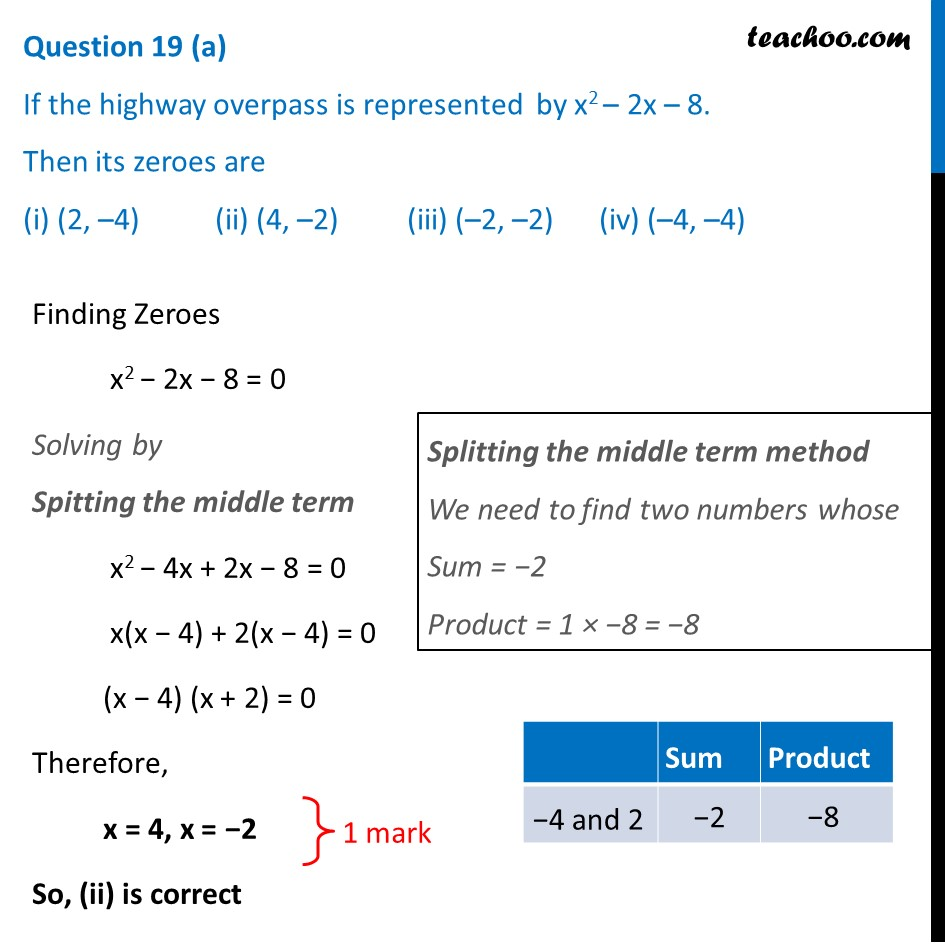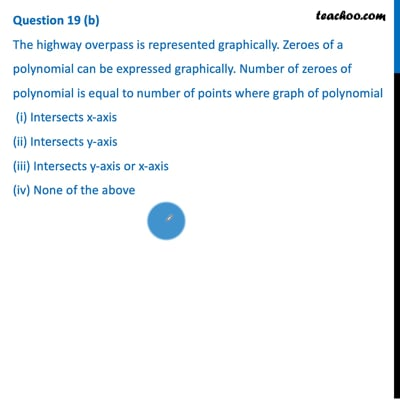This video is only available for Teachoo black users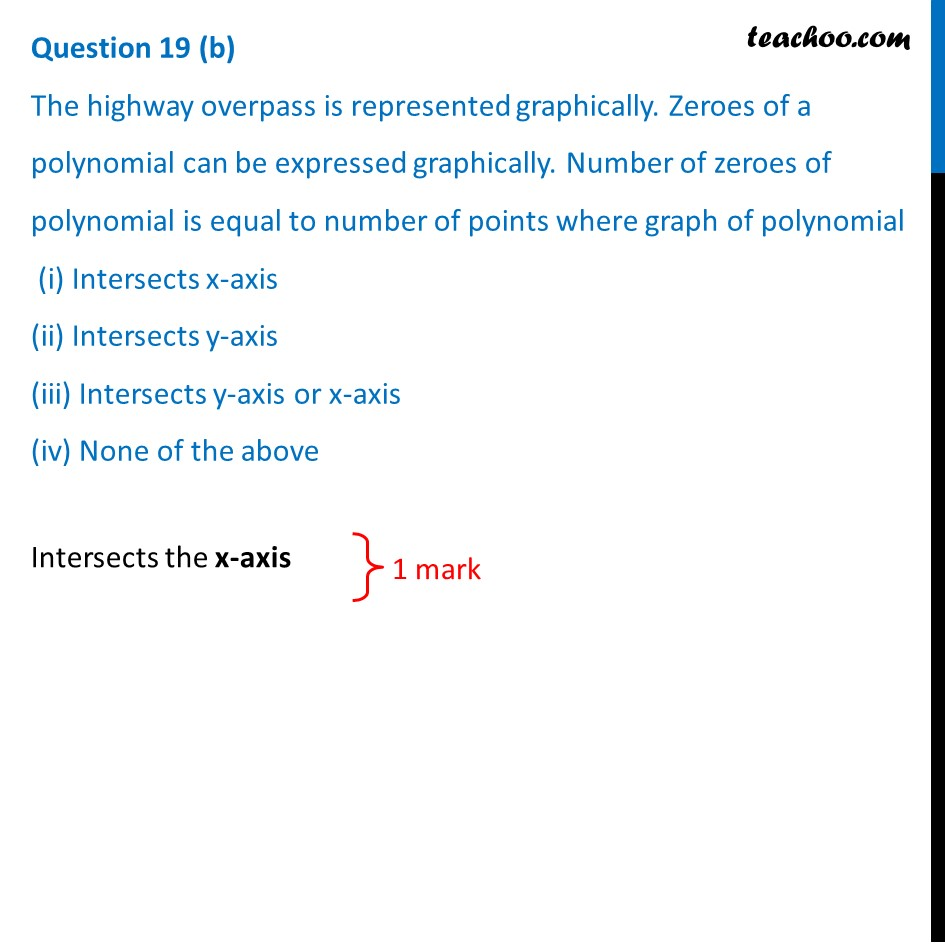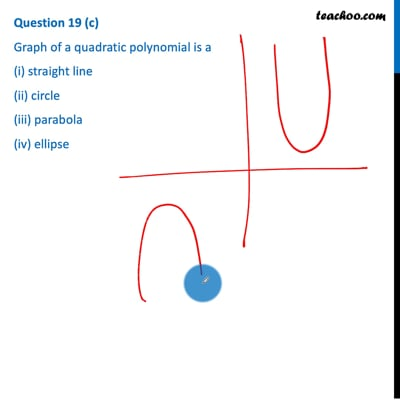This video is only available for Teachoo black users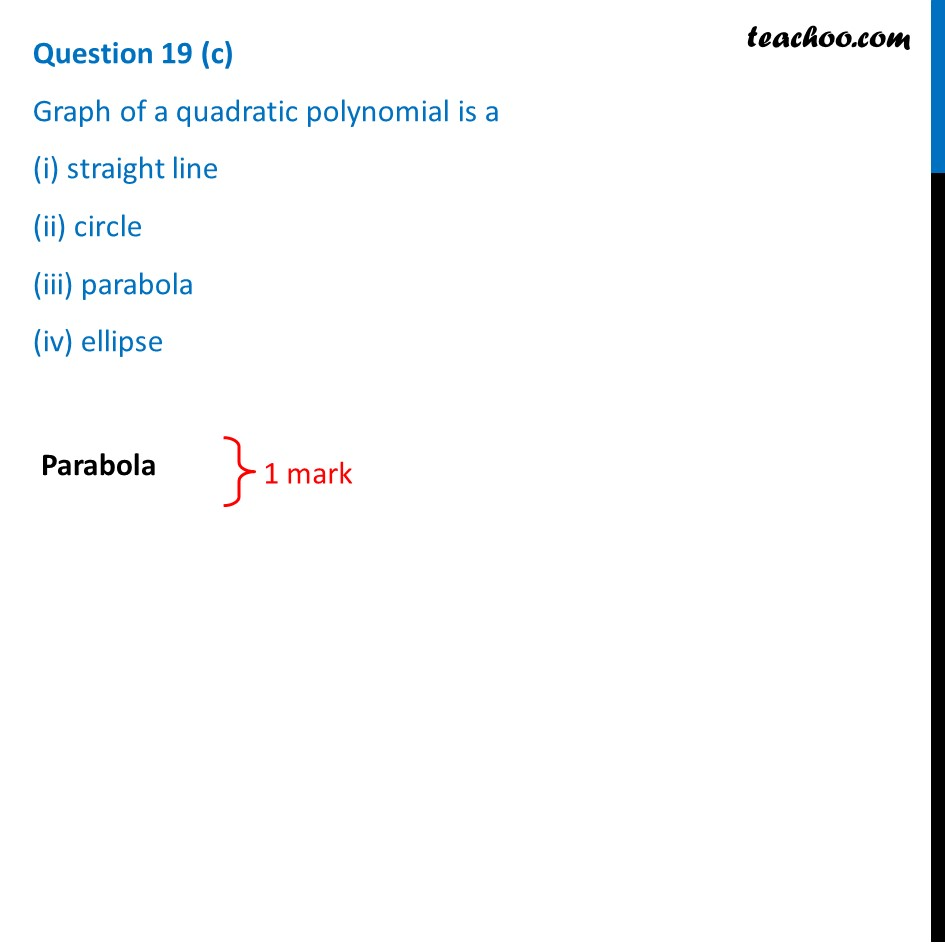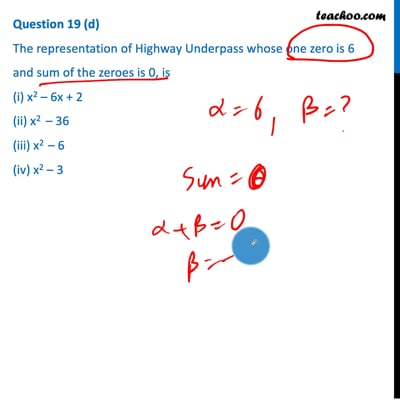This video is only available for Teachoo black users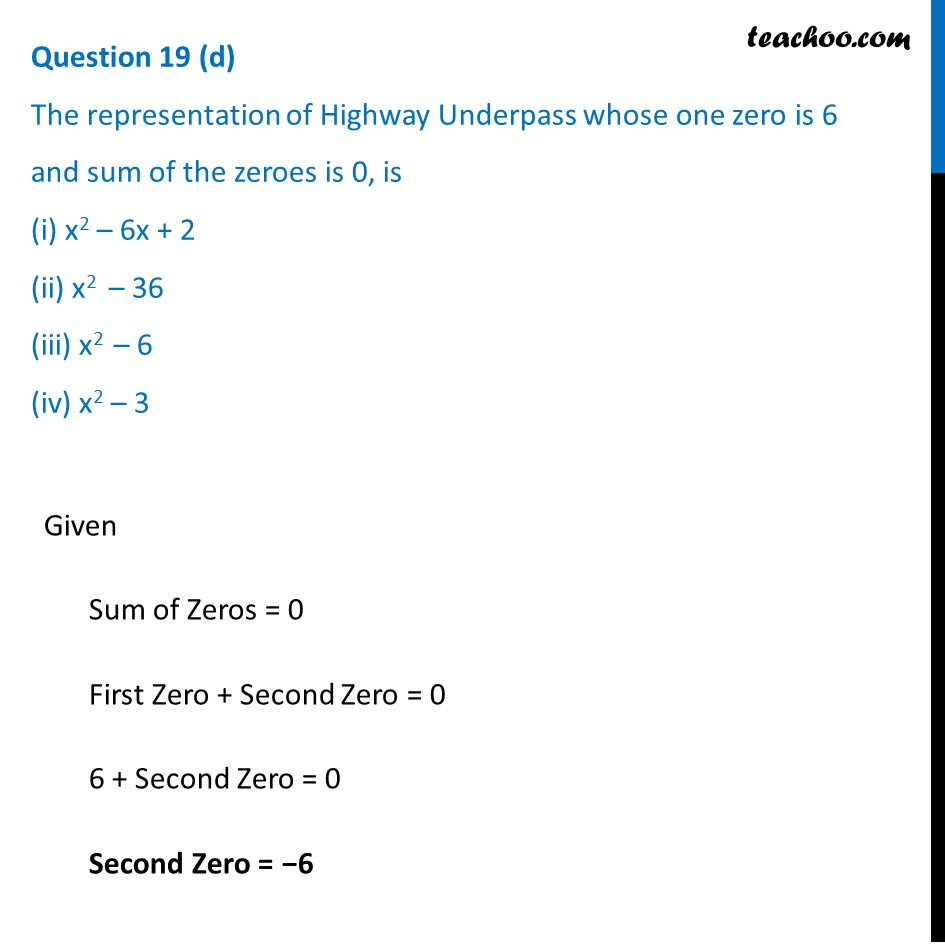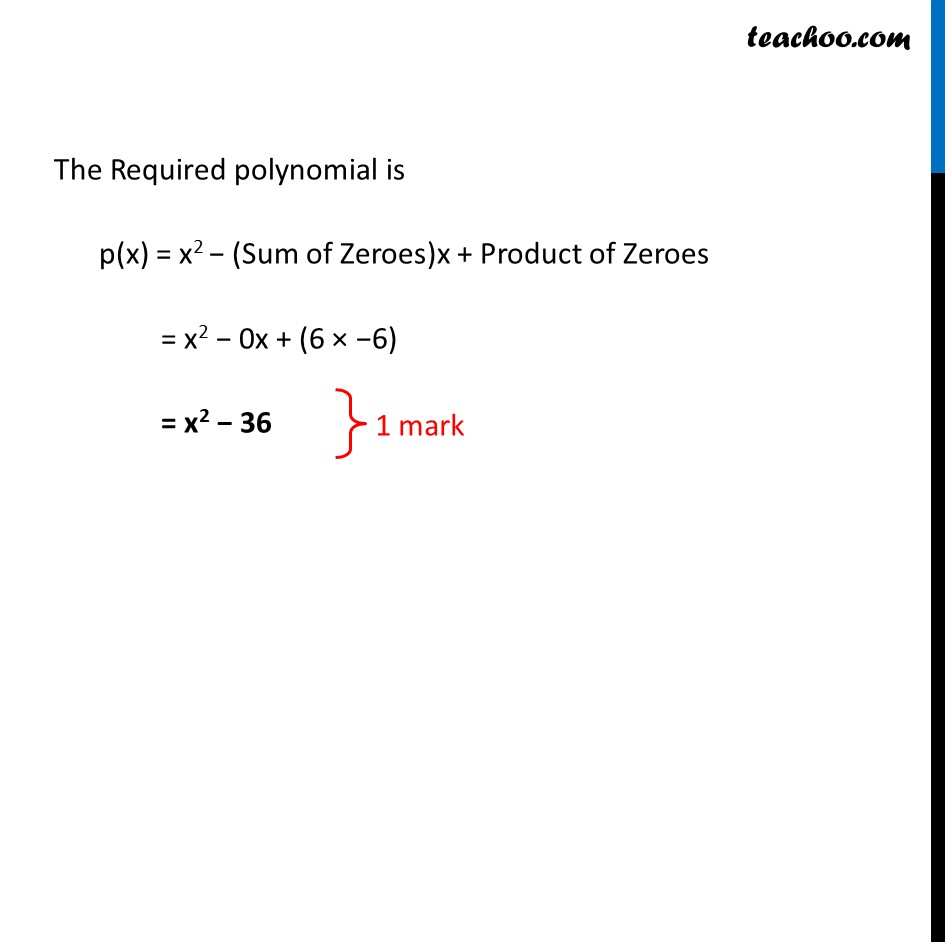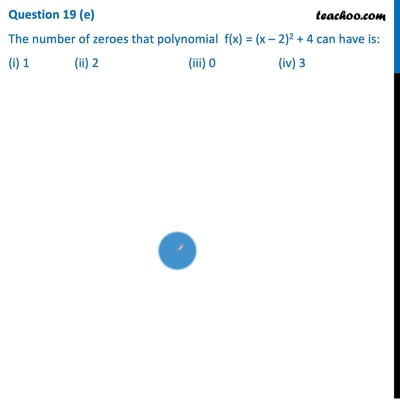This video is only available for Teachoo black users

Note: Check more Case Based Questions for Polynomials Class 10

Introducing your new favourite teacher - Teachoo Black, at only ₹83 per month

### Transcript

Question 19 Case Study Based- 3 Applications of Parabolas-Highway Overpasses/Underpasses A highway underpass is parabolic in shape.Parabola A parabola is the graph that results from p(x)= ax2 + bx + c Parabolas are symmetric about a vertical line known as the Axis of Symmetry. The Axis of Symmetry runs through the maximum or minimum point of the parabola which is called the Vertex (a) If the highway overpass is represented by x2 – 2x – 8. Then its zeroes are (i) (2, –4) (ii) (4, –2) (iii) (–2, –2) (iv) (–4, –4) Finding Zeroes x2 − 2x − 8 = 0 Solving by Spitting the middle term x2 − 4x + 2x − 8 = 0 x(x − 4) + 2(x − 4) = 0 (x − 4) (x + 2) = 0 Therefore, x = 4, x = −2 So, (ii) is correct (b) The highway overpass is represented graphically. Zeroes of a polynomial can be expressed graphically. Number of zeroes of polynomial is equal to number of points where graph of polynomial (i) Intersects x-axis (ii) Intersects y-axis (iii) Intersects y-axis or x-axis (iv) None of the aboveIntersects the x-axis (c) Graph of a quadratic polynomial is a (i) straight line (ii) circle (iii) parabola (iv) ellipseParabola (d) The representation of Highway Underpass whose one zero is 6 and sum of the zeroes is 0, is (i) x2 – 6x + 2 (ii) x2 – 36 (iii) x2 – 6 (iv) x2 – 3Given Sum of Zeros = 0 First Zero + Second Zero = 0 6 + Second Zero = 0 Second Zero = −6 The Required polynomial is p(x) = x2 − (Sum of Zeroes)x + Product of Zeroes = x2 − 0x + (6 × −6) = x2 − 36 (e) The number of zeroes that polynomial f(x) = (x – 2)2 + 4 can have is: (i) 1 (ii) 2 (iii) 0 (iv) 3 To find Zero, We put f(x) = 0 and find value of x Putting f(x) = 0 (x – 2)2 + 4 = 0 (x – 2)2 = −4 Since Square cannot be negative This is not possible Therefore, this Polynomial doesn’t have a Zero Number of Zeros = 0